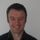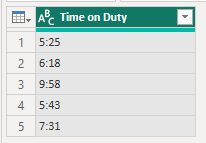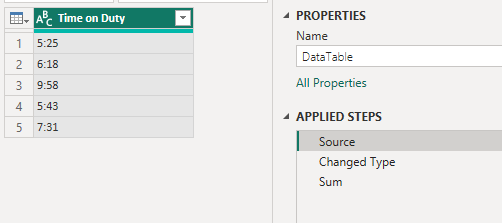cancel
Showing results for
Did you mean:## Sum Hours and Minutes

Hi,  I have a simple column for hours and minutes as below. How can I sum it and display results in hours and minutes? I've searched all these solutions and just not able to find anything that simply sums them up and gives a total in hours and minutes.

Time on Duty

5:25

6:18

9:58

5:43

7:31

2 ACCEPTED SOLUTIONSSuper User

Unfortunately this isn't as straight forward as you'd think.Looking at your data I'd say it's text.  So you first need to convert that to Duration.

Now you have a column of durations and there's no simple way to sum these.  So you have to write some custom code in the Advanced Editor.

You end up with a single value.  You haven't specified how you want the sum presented so for now, I'll leave it as that, but you can have it stored other ways if you need to use it in subsequent steps, calculatiosn etc.

``````let
Source = Table.FromRows(Json.Document(Binary.Decompress(Binary.FromText("i45WMrUyMlWK1YlWMrMytAAzLK1MIQxTKxNjMMPcythQKTYWAA==", BinaryEncoding.Base64), Compression.Deflate)), let _t = ((type nullable text) meta [Serialized.Text = true]) in type table [#"Time on Duty" = _t]),
#"Changed Type" = Table.TransformColumnTypes(Source,{{"Time on Duty", type duration}}),
Sum = List.Sum(#"Changed Type"[Time on Duty])
in
Sum``````regards

Phil

If I helped you, click on the Thumbs Up to give Kudos.

Proud to be a Super User!Super User

You're only seeing the result of all applied steps in Power Query, but if you click on the other steps, you'll see the original source table in the first step called Source. Then the data converted to Durations in the Changed Type step.To show the duration in hours you can use the Duration.TotalHours function on the Sum I calculated in my first attempt.

In the attached file I've duplicated my first query then written an extra line of code to give you the decimal total of 34.91666666 hours.  If you want to round that to 34.92 you can wrap it in Number.Round().

I'm getting the feeling that you want to see the Time on Duty column and the total time worked at the same time.  Because of the way Power Query works, using columns and rows to store data, and because you are asking for a single value as a result, how would you want a single value displayed in a table of columns and rows?

One way would be to have a query in Power Query that holds the Time on Duty column, and a separate table that holds the total time worked.

You can display both of these separately in Power BI visuals - see attached file.

Note that what is displayed in the visual depends on the data type brought into Power BI.  If you leave things as text, it might be easier to read, but you can't do any calculations with text.

If you convert the data to durations then these are displayed as decimals (parts of a day), which might be harder to understand, but you can do calculations with these.

BTW, you mention DAX, but this is done in Power Query.  It is possible in DAX but is even more convoluted.  I wrote about it here

Convert Decimal time to days, hours, minutes, seconds in DAX

regards

Phil

If I helped you, click on the Thumbs Up to give Kudos.

Proud to be a Super User!

4 REPLIES 4Super User

You're only seeing the result of all applied steps in Power Query, but if you click on the other steps, you'll see the original source table in the first step called Source. Then the data converted to Durations in the Changed Type step.To show the duration in hours you can use the Duration.TotalHours function on the Sum I calculated in my first attempt.

In the attached file I've duplicated my first query then written an extra line of code to give you the decimal total of 34.91666666 hours.  If you want to round that to 34.92 you can wrap it in Number.Round().

I'm getting the feeling that you want to see the Time on Duty column and the total time worked at the same time.  Because of the way Power Query works, using columns and rows to store data, and because you are asking for a single value as a result, how would you want a single value displayed in a table of columns and rows?

One way would be to have a query in Power Query that holds the Time on Duty column, and a separate table that holds the total time worked.

You can display both of these separately in Power BI visuals - see attached file.

Note that what is displayed in the visual depends on the data type brought into Power BI.  If you leave things as text, it might be easier to read, but you can't do any calculations with text.

If you convert the data to durations then these are displayed as decimals (parts of a day), which might be harder to understand, but you can do calculations with these.

BTW, you mention DAX, but this is done in Power Query.  It is possible in DAX but is even more convoluted.  I wrote about it here

Convert Decimal time to days, hours, minutes, seconds in DAX

regards

Phil

If I helped you, click on the Thumbs Up to give Kudos.

Proud to be a Super User!Thank you, Phil, your approach is very helpful and I was able to arrive at what I needed with your help. I basically have the time on duty in the data as displayed in original post. I changed the data type into duration, in which case Power Query turns it into decimal in terms of days. So to turn it into hours, I created a measure multiplying these falues by 24. So that gave me total hours on duty.

Thank you so much for your hlep.Super User

Unfortunately this isn't as straight forward as you'd think.Looking at your data I'd say it's text.  So you first need to convert that to Duration.

Now you have a column of durations and there's no simple way to sum these.  So you have to write some custom code in the Advanced Editor.

You end up with a single value.  You haven't specified how you want the sum presented so for now, I'll leave it as that, but you can have it stored other ways if you need to use it in subsequent steps, calculatiosn etc.

``````let
Source = Table.FromRows(Json.Document(Binary.Decompress(Binary.FromText("i45WMrUyMlWK1YlWMrMytAAzLK1MIQxTKxNjMMPcythQKTYWAA==", BinaryEncoding.Base64), Compression.Deflate)), let _t = ((type nullable text) meta [Serialized.Text = true]) in type table [#"Time on Duty" = _t]),
#"Changed Type" = Table.TransformColumnTypes(Source,{{"Time on Duty", type duration}}),
Sum = List.Sum(#"Changed Type"[Time on Duty])
in
Sum``````regards

Phil

If I helped you, click on the Thumbs Up to give Kudos.

Proud to be a Super User!Thank you, Phil. I'm having a little trouble following your solution as the table with the hour and minute values you have posted is not in the PBX file. The PBX file only has the final sum.

I would like the total to be represented in hours and minutes (not days). So the total for this example would be 34 hours and 55 minutes. I would then like to convert this into decimals so it would be 34.92 hours.

Any way you would be able to include the Time On Duty column in the PBX file along with the code you wrote so I can see how this was done step by step?

Sorry, I'm not that good with DAX so would like to follow along the example to replicate in my data.

Right in the PBX file you shared, when I click on Data View, all I'm seeing is below:

DataTable

1.454861111